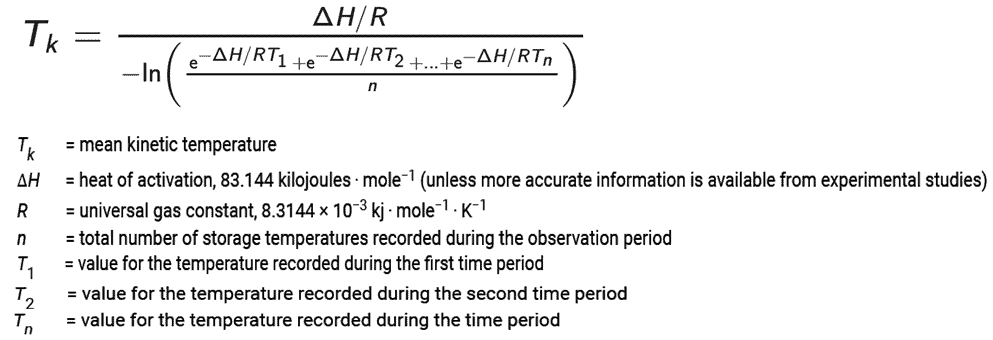# Online Mean Kinetic Temperature (MKT) Calculator

## What is Mean Kinetic Temperature (MKT)

Mean Kinetic Temperature (MKT) is a single derived temperature that, if sustained over a specified time, yields the same thermal challenge to a drug product or drug substance as would be experienced over a range of higher and lower temperatures for an equivalent period. The Mean Kinetic Temperature (MKT) is higher than the arithmetic mean temperature.

Mean Kinetic Temperature (MKT) is calculated according to the Arrhenius equation.

When establishing the mean kinetic temperature for a defined period, the formula of J. D. Haynes (J. Pharm. Sci., 60:927- 929, 1971) can be used.

Reference: ICH Q1A (R2) “Stability Testing of New Drug Substances & Products”

## The Mean Kinetic Temperature (MKT) calculation equation is as follows.Looking at the equation looks complicated; however, you can follow the steps to calculate Mean Kinetic Temperature (MKT) using spreadsheets such as excel sheet or google docs.
The step-by-step process to calculate MKT is as follows:
Step 1: Convert the temperature from °C to Kelvin (°K) by adding 273.15 to each reading.
Step 2: Calculate [Delta H/(Gas Constant x Temperature Reading)]. Where, Delta H is 83.14472 kJ/ mole and Gas Constant = 0.008314472 kJ/ mole/ degree.
Step 3: Calculate the total of all results that are derived from Step 2.
Step 4: Divide the value achieved in Step 3 by the number of readings.
Step 5: Calculate the NL (Natural Log) of the result derived in Step 4.
Step 6: Calculate the numerator Delta H divided by Gas Constant.
Step 7: Calculate the result derived in Step 5 by the result of Step 6.
Step 8: Convert Kelvin (°K) to °C. The achieved value is the Mean Kinetic Temperature (MKT) value.

Still its complicated, feel free to use this online Mean Kinetic Temperature (MKT) calculator or you also can download the Free Excel Template Sheet for calculating Mean Kinetic Temperature (MKT). Click Here to get the free excel sheet for MKT Calculation.